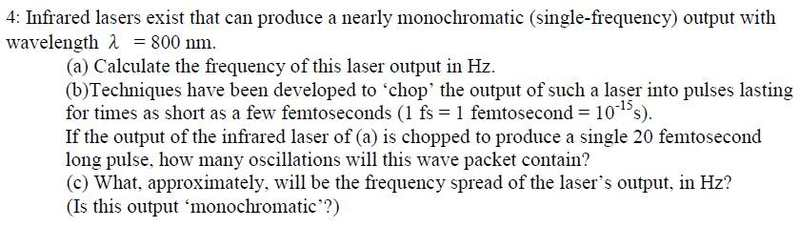# Wavelength/Frequency short Questions help

mmmbohFor a) I did frequency = speed of light / wavelength = 3.75x1014Hz
for b) I did 3.75x1014Hz x 10-15s=0.375
I think a) is right, but I don't know about b)
For c) I would have thought it is the same frequency as the first because I am taking it as if it is the same frequency, but a shorter amount of time, but I think this is wrong.

Help?

mmmboh

Homework HelperFor a) I did frequency = speed of light / wavelength = 3.75x1014Hz
for b) I did 3.75x1014Hz x 10-15s=0.375

10^-15 s is one femtosecond, not 20.

For c) I would have thought it is the same frequency as the first because I am taking it as if it is the same frequency, but a shorter amount of time, but I think this is wrong.

Have you tried the energy-time uncertainty principle?

mmmboh
Oh right, so I guess b) would be what I put times 20, so 7.5...
c) If that has anything to do with heisenberg's uncertainty principle, we haven't been taught that in this class, is there another way?

mmmboh
Or if I use that which equation should I use? I looked at it, but I'm not sure how it applies.

mmmboh
Hm I looked at the equations, and have found that $$1/2\delta T = 2.5x10^{13}hz$$ where delta T = 20 femtoseconds...is this correct?